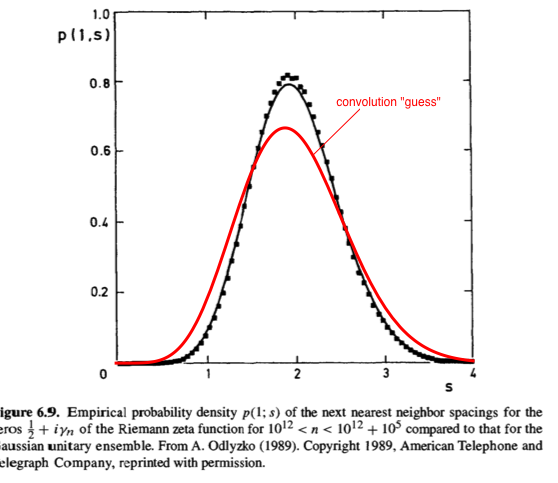# Riemann zeta function: pair correlations vs. neighbor spacings

Montgomery's pair correlation conjecture states that the distribution of the pair correlations of the zeroes of the Riemann zeta function (normalized to have average spacing 1) is given by the function $$g(u) = 1 - \left(\frac{\sin(\pi u)}{\pi u}\right)^2 + \delta(u).$$ See https://en.wikipedia.org/wiki/Pair_correlation_conjecture for some basic information. Famously, this distribution also appears in random matrix theory.

There is also a conjecture for the distribution of the nearest neighbor spacings of the normalized zeroes, which is conjectured to be given by $$f(u) = \frac{32}{\pi^2} u^2 \exp\left(-\frac{4}{\pi} u^2 \right)$$

Question: What is the conjectured distribution of the second-nearest neighbors? Can it be obtained by assuming that consecutive spacings are stochastically independent? - if "yes", then we could obtain the conjectured distribution $f_2(u)$ of the spacings of the second-nearest neighbors by convolution, that is, by the formula $f_2(u) = (f*f) (u)$. Does it work this way?

Furtheremore, writing $f^{*k}$ for $\underbrace{f* \dots * f}_{k \textrm{ times}}$ - then if all consecutive spacings were conjectured to be independent, we should have $$g(u) = \delta(u) + \sum_{k=1}^\infty f^{*k}(u).$$ Is this last equality true? (I don't know how to check it.)

• Perhaps you could try to expand $g(u)$ as a Taylor series, and then perform a Fourier transform on each summand. Feb 1 '16 at 10:49
• I tried a little bit, but couldn't get far - it was quite messy. Note that $f(u)$ is actually the density of a probability distribution, but $g(u)$ is not. In particular, $g(u) \to 1$ as $u \to \infty$, so it's not possible to capture it directly using Fourier transforms. Feb 1 '16 at 11:17
• The function you quote for $f(u)$ is called the Wigner surmise. It is exact only for $2\times 2$ random matrices. The $N\to\infty$ asymptotic of the $N\times N$ result, which would apply to the zeta function, is much more complicated. Feb 1 '16 at 11:59
• @Marcel --- but the difference between the Wigner surmise and the exact large-$N$ result is tiny (a few percent), isn't it? Feb 1 '16 at 12:09
• @Marcel: thanks, I was not aware of that. Where can I find a formula for the limit distribution? And, more importantly, how about the question concerning convolutions? Feb 1 '16 at 12:11

This next-nearest-neighbor distribution of the Riemann zero's is addressed in Mehta's book on random-matrix theory. It is well reproduced by that of the Gaussian Unitary Ensemble (GUE), compare black curve and black data points:I added the red curve in the plot, being the convolution of the nearest-spacing distribution in the GUE:

$$p(s)=\int_0^s f(u)f(s-u)du,\;\;\text{with}\;\;f(u) = \frac{32}{\pi^2} u^2 \exp\left(-\frac{4}{\pi} u^2 \right)$$

As you can see, it is quite different, so this "independent spacing" assumption is not reliable.

All of this is for complex matrices (GUE). For real symmetric matrices (GOE) the distribution of next-nearest-neighbor spacings is given by the distribution of the nearest-neighbor spacings of quaternion Hermitian matrices (GSE).

• Hi Carlo, this is very interesting, thank you. According to your comment above this deviation also does not come from the fact that instead of $f(u)$ we should take a slightly different distribution, but rather shows that there is something genuinely wrong with assuming that consecutive spacings are independent. Feb 1 '16 at 12:52
• PS: For me the interpretation of your picture is that consecutive spacings are not independent, but that rather a short spacing is more likely to be followed by a larger spacing (to "make up for it") and vice versa. Nice. Feb 1 '16 at 13:11
• indeed, the rigidity of the spectrum anticorrelates the fluctuations in the spacings of subsequent eigenvalues; the difference between the Wigner surmise and the exact spacing distribution is hardly noticeable on the scale of this figure. Feb 1 '16 at 16:29